1.5 Division method for finding square root:

We have learnt how to find the square root of a perfect number by factorization method, where we list all the factors of perfect numbers and then take square root of the factors

Ex : 484 = 2*2*11*11 = 22*112

Therefore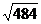= 2*11

Factorisation method is time consuming when the number whose square root to be found, is too large. Because of this reason we follow another method called division method to find the square roots.

In this method we pair the digits whose square root has to be found, from the right side (units place).

If the number of digits in the number is even then all the groups will have 2 digits.

For example the number  219024 is grouped into three pairs of (21),(90) and (24).

The number  34567890   is grouped as (34,56,78,90).

If the number of digits in the number is odd then the first group will have one digit and rest will have two digits.

For example the number 19024  is grouped in to three groups of (1),(90) and(24).

Similarly 3456789 is grouped as (3),(45),(67) and (89).

1.5.1 Finding square root of whole numbers:

1.5.1 Problem 1: Find square root of 219024 by division method

Solution :

Step1 : Group  the pair of digits from right side(unit place). The three groups are 21,90,24.

Step2 :  Find the Largest  square number less than or equal to  the first group (21).

Since 52>21 and 42<21. 16 is the number.

Step 3 : Take the square root of 16 = 4

Step 4:  Place 4 as quotient above the first group,

Step 5 : Place 4 also as divisor

Step 6 : Subtract from the first group (21), the product of  divisor and quotient=16(=4*4) :The reminder is 5(21-16).

Step 7 : Consider this remainder and the  second group (=590) as the new dividend.

Step 8 : Add divisor and the digit in the unit place of divisor.(4+4= 8). Find a digit x such that 8x multiplied by itself(x) gives a number < or = the new dividend (=590). We find that 86*6 = 516 which is less than the dividend 590. Therefore x=6 . 86 will be the new divisor. Write 6 above the second group at the top.

Step 9 : Subtract 516, the product of this new divisor(86) and 6 from the dividend 590 - 86 *6 =74

Step 10: Consider this remainder (74) and the next group (24) as the new dividend (7424)

Step 11: Add divisor and the digit in the unit place of divisor.( 86+ 6= 92).Find a digit x such that 92x multiplied by itself(x) gives a number < or = the new dividend (=7424). We find that 928*8 = 7424 which is equal to dividend. Therefore x=8. Write 8 as quotient above the third group at the top.

Step 12 : Subtract 7424, the product of this new divisor(928) and the quotient  8  from the dividend 7424 -928*8 =0

Step 13 : Continue this process till  there  are no more groups for division.

We stop the process here as there are no more groups remaining for division.

 Step Divisor 4     6    8 Explanation 2,3,5 4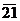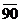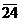16<21<25 ,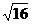=4 6 +4 16 16=4*4 7,8 86 590 21-16=5, 8 =4+4 9 +6 516 86*6 =516 10,11 928 7424 86+6 =92: 590-516 =74 12 7424 928*8=7424 0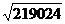= 468

Verification:

468 is of the form460+8 and we know the identity (a+b)2=a2+2ab+b24682=4602+2*460*8+82

= 211600+7360+64 = 219024

1.5.1  Problem 2: Find square root of 657721 by division method

Solution :

 Step Divisor 8    1    1 Explanation 2,3,5 8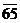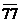64<65<81 ,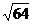=8 6 +8 64 64=8*8 7,8 161 1 77 65-64=1 16 =8+8 9 +1 161 161*1 =161 10,11 1621 1621 161+1 =162: 177-161=16 12 1621 1621*1 =1621 0

We stop the process here as there are no more groups remaining for division.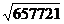= 811

Verification:

811 is of the form800+10+1 and we know the identity (a+b+c)2=a2+b2+c2+2(ab+bc+ca)

8182= 8002+102+12+2(800*10+10*1+800*1)

= 640000+100+1+2*(8000+10+800) = 640000+101+17620 =657721

1.5.1  Problem 3: Find square root of 49244 by division method

Solution :

Since the number has odd digits, there will be only one number (4) in the first group

 Step Divisor 2    2   2 Explanation 2,3,5 2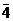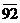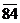4=4<9 ,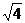=2 6 +2 4 4=2*2 7,8 42 0 92 4-4=0 4 =2+2 9 +2 84 42*2 =84 10,11 442 884 42+2 =44: 92-84 =8 12 884 442*2 =884 0

We stop the process here as there are no more groups remaining for division.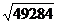= 222

Verification:

222 is of the form200+20+2 and we know the identity (a+b+c)2=a2+b2+c2+2(ab+bc+ca)

2222= 2002+202+22+2(200*20+20*2+200*2)

= 40000+400+4+2*(4000+40+400) = 40000+404+8480 =49284

1.5.1  Problem 4 :  A Gardner arranges the 64014 plants in rows to form a square. In doing so, he finds he is left with 5 plants. Find the number of plants in each row.

Solution:

Since, the gardener is left with 5 plants after arranging in rows. Number of plants used for planting

=64009 (=64014-5).

Because the plants are planted in rows of perfect square, we need to find the square root of 64009.

Since 64009 has odd number of digits, there will be only one number (6) in the first group and other groups are (40) and (09).

 Step Divisor 2    5   3 Explanation 2,3,5 2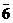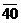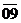4=4<6 ,=2 6 +2 4 4=2*2 7,8 45 2 40 6-4=2 4 =2+2 9 +5 2 25 45*5 =225 10,11 503 15 09 45+5 =50: 240-225 =15 12 884 503*3 =1509 0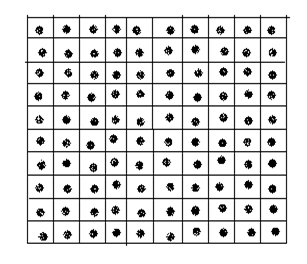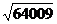= 253

Hence the gardener had arranged the roses in 253 rows with 253 plants in each row

Verfication:

253 is of the form250+3 and we know the identity (a+b)2=a2+2ab+b2

2532= (250+3)2= (250)2+2*250*3+(3)2

= 62500+1500+9 = 64009

1.5.1  Problem 6 :  Find the least number which must be added to  9215 to make it a perfect square.

Solution:

 Step Divisor 9    6 Explanation 2,3,5 9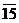81<92<100 ,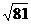=9 6 +9 81 81=9*9 7,8 186 11 15 92-81=11 18 =9+9 +6 11 16 185*5 =925,186*6 =1116 -1 1115-1116 = -1

We stop here as there are no more groups to be considered.

We have seen that in case of perfect numbers, the reminder in the last step has to be zero, which was not the case in the above problem.

Since it was given that 9215 is less than the nearest perfect square, in the last step we had to have a reminder.

9215+1 is a perfect square and the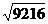= 96

Verfication:

Verify that 962= 9216

1.5.1  Problem 7:  Find the least number which must be subtracted  from 5084  to make it a perfect square.

Solution:

 Step Divisor 7    1 Explanation 2,3,5 7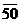49<50<64 ,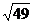=7 6 +7 49 81=9*9 7,8 141 1 84 92-81=11 18 =9+9 +1 1 41 141*1 =141,141*2 =282 43 184-141=43

We stop here as there are no more groups to be considered.

We have seen that in case of perfect numbers, the reminder in the last step has to be zero, which was not the case in the above problem.

Since it was given that 5084 is larger  than a perfect square, in the last step, we had to have a reminder.

5041= 5084-43 is a perfect square and thus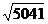= 71

Verfication:

Verify that 712= 5041

Observations:

 Number Its Square 3(1 digit) 9(1 digit) 4(1 digit) 16(2 digits) 31(2 digits) 961(3 digits) 32(2 digits) 1024(4 digits) 316(3 digits) 99856(5 digits) 317(3 digits) 100489(6 digits) 3162( 4 digits) 9998244(7 digits) 3163(4 digits) 10004569(8 digits) .3(1 place after decimal) .09( 2 places after decimal) .01(2 places after decimal) .0001(4 places after decimal) .001(3 places after decimal) .000001( 6 places after decimal)

Conclusion: If a number has n digits, then its square root will have n/2 digits if n is even and (n+1)/2 digits if n is odd. If a number has n decimal places after it, then its square root will have n/2 digits after decimal places.

1.5.2 Finding the square root of decimals:

We follow the same procedure that we followed in the case of whole numbers. The main difference is the way we form groups. In case of decimal numbers:

The grouping of whole numbers is done from the left side in to groups of two numbers. In the case of numbers after the decimal point, we form groups of two numbers to the right of the decimal number.

Ex: the grouping for 205.9225 is done as (2), (05), (92), (25)

The division process is separately carried out for whole numbers and decimal numbers.

1.5.2 Problem 1: Find square root of  235.3156 by division method

Solution :

We group 2 and 35 as groups for whole numbers

We group 31 and 56 as groups for decimal numbers

 Step Divisor 1  5.  3 4 Explanation 2,3,5 1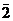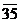.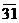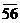1<2<9 ,=2 6 +1 1 1=1*1 7,8 25 1 35 2-1=1 2 =1+1 9 +5 1  25 25*5 =125 10,11 303 1031 255+5 =30: 135-125 =10.Put a decimal point at the top after 15 as we have started taking groups from decimal part 12 +3 909 303*3 =909 3064 122 56 303+3=306 122  56 3064*4 =12256 0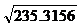= 15.34

Verfication:

Verify that 15.342= 235.3156

ALTERNATE METHOD:

First, follow the division method to arrive at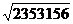=1534. We know that 235.3156 = 2353156/10000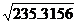=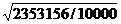=/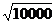=/100 = 1534/100 = 15.34

Finding square root of numbers which are not perfect squares.

Any number x  is same as x.0000

( 5 is same as 5.0000 and 11 = 11.0000)

In order to find the square root of a non perfect number we rewrite that number as the same number with four zeros after the decimal point and we then follow division method to find the square root of that number with four decimals.

1.5.2 Problem 2: Find the approximate length to 3 decimal places  of the side of a square whose area is 12.0068 sq. meters

Since we are required to find the length to 3 decimal places, we need to rewrite the number with 6 decimals.

Solution :

12.0068 = 12.006800

The group for whole number is 12 and groups for decimal parts are (00), (68),and (00)

 Step Divisor 3 .  4   6  5 Explanation 2,3,5 3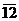.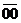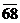9<12<16 ,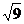=3 6 +3 9 3*3=9 7,8 64 3   00 12-9=3 6 =3+3 Put a decimal point at the top after 3 as we have started taking groups from decimal part 9 +4 2  56 64*4 =256 10,11 686 44 68 64+4 =68:300-256=44, take next group 12 +6 41 16 686*6 =4116 6925 3  52 00 686+6=692 3  46 25 6925*5 =34625 5 75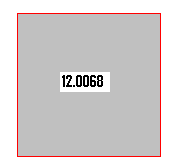We may continue this division method to the required number of decimal points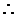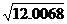is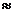3.465 rounded to 3.47Verfication:

Verify that 4.4652= 12.006225

You may notice that the value of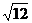to 14 decimal places is = 3.46410161513775

Since 12 is not a perfect square the decimals inis never ending.

Note: if it is required to find the square root of rational number, then convert the number in to a decimal number and follow the procedure as given above(for example to find the square root of 11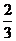, convert this number to decimal as 11.6666 and then follow the procedure as in 1.5.2 Problem 2)

Alternatively convert the rational number in to a number free from radical sign in the denominator by suitable multiplication and then calculate the square root of numerator as worked out earlier.

For example 11=35/3 = (35*3) ÷ (3*3) = 105÷9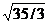=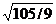= (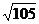)/3

1.5 Summary of learning

 No Points studied 1 2 Finding square root by division method Finding square root of decimals